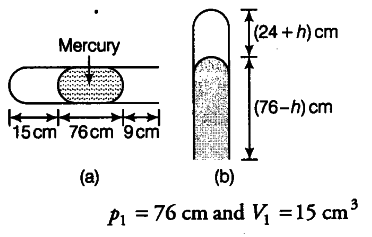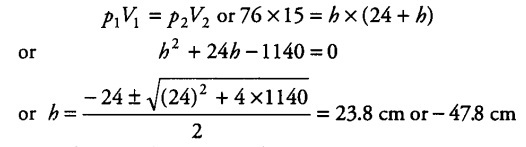# A meter long narrow bore held horizontally

A meter long narrow bore held horizontally (and closed at one end) contains a 76 cm long mercury thread which traps a 15 cm column of air. What happens, if the tube is held vertically with the open end at the bottom?

If the tube is held horizontally, the mercury thread of length 76 cm traps a length of air = 15 cm. A length of 9 cm of the tube will be at the open end, Fig. (a). The pressure of air enclosed in tube will be atmospheric pressure. Let area of cross-section of the tube be 1 sq. cm.If the tube is held vertically, 15 cm air gets another 9 cm of air (filled in the right handside in the horizontal position) and let h cm of mercury flow out to balance the atmospheric pressure, Fig. (b). Then, the heights of air column and mercury column are {24 + h) cm and (76 - h) cm respectively.
The pressure of air =76(76-h) = h cm of mercury
{ V }_{ 2 } = (24+h) {{cm}^{3}} and
{ p }_{ 2 } = h cm
If we assume that temperature remains constant, thenSince h cannot be negative (because more mercury cannot flow into the tube), therefore h = 23.8 cm. Thus, in the vertical position of the tube, 23.8 cm of mercury flows out.